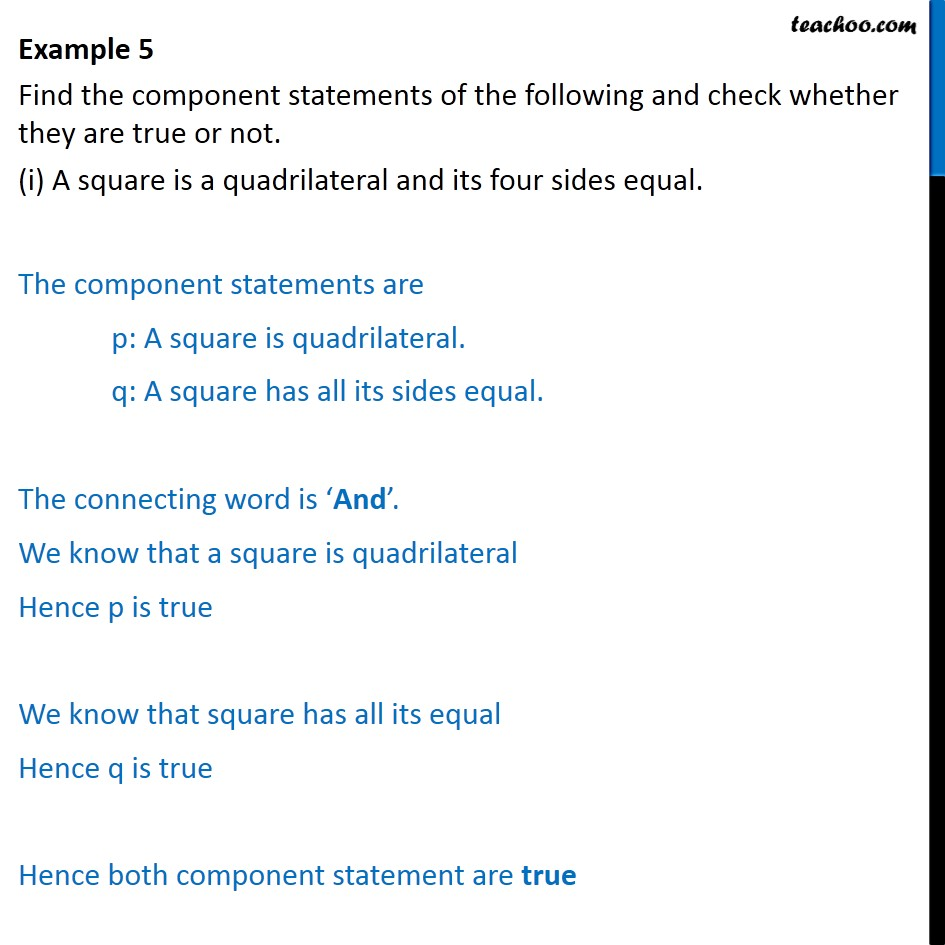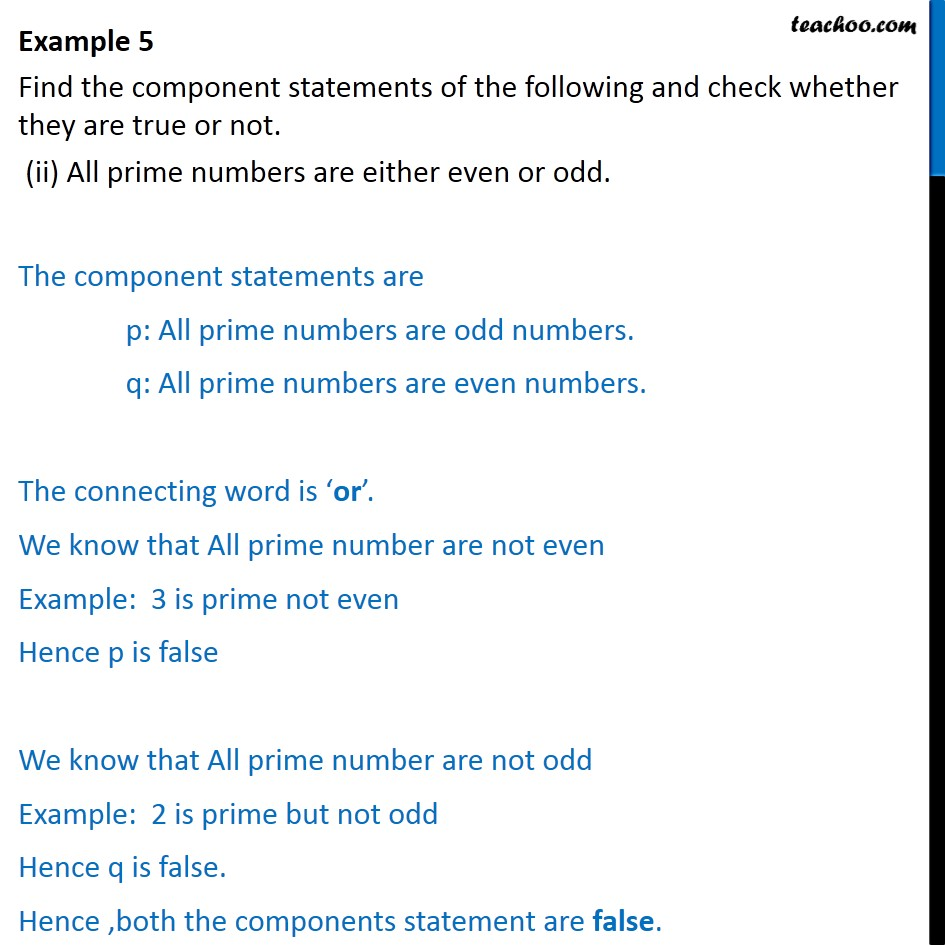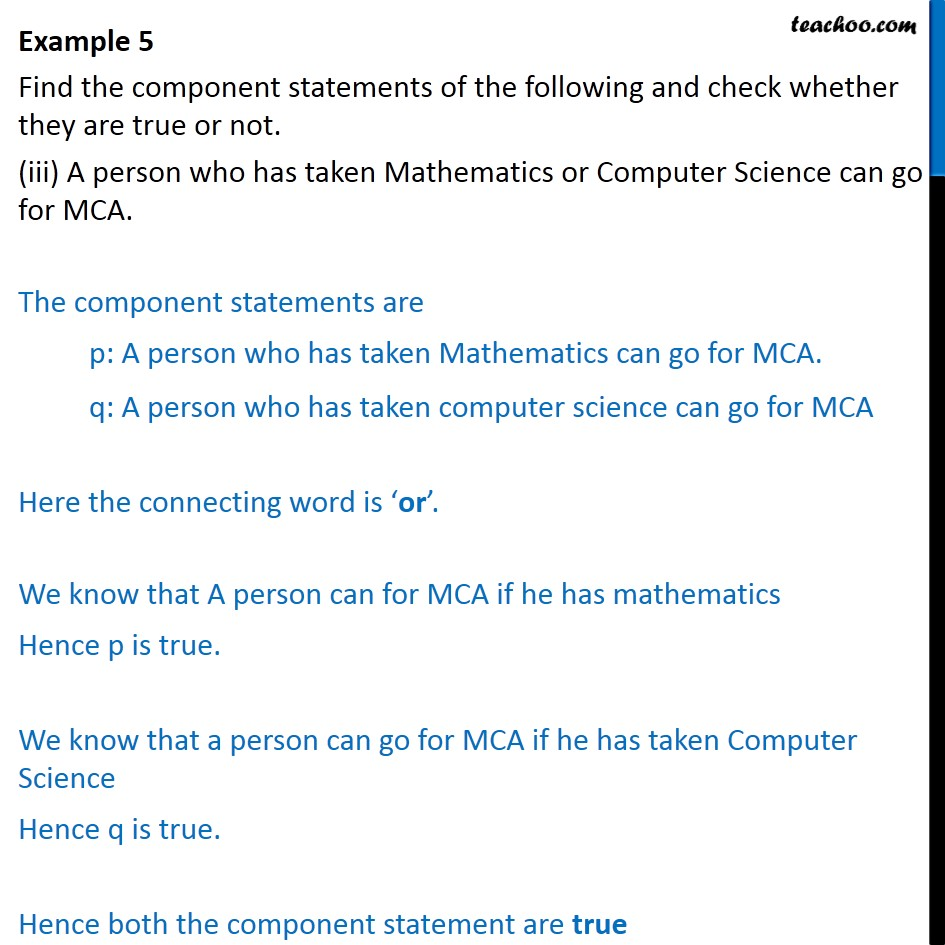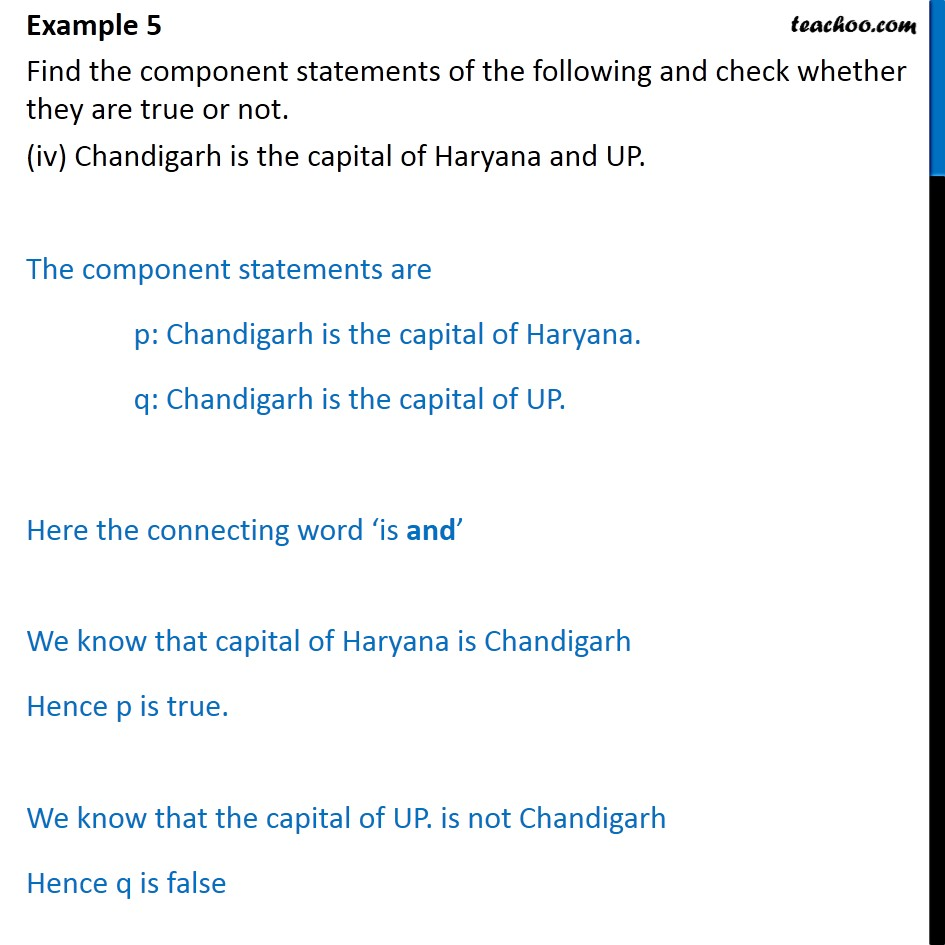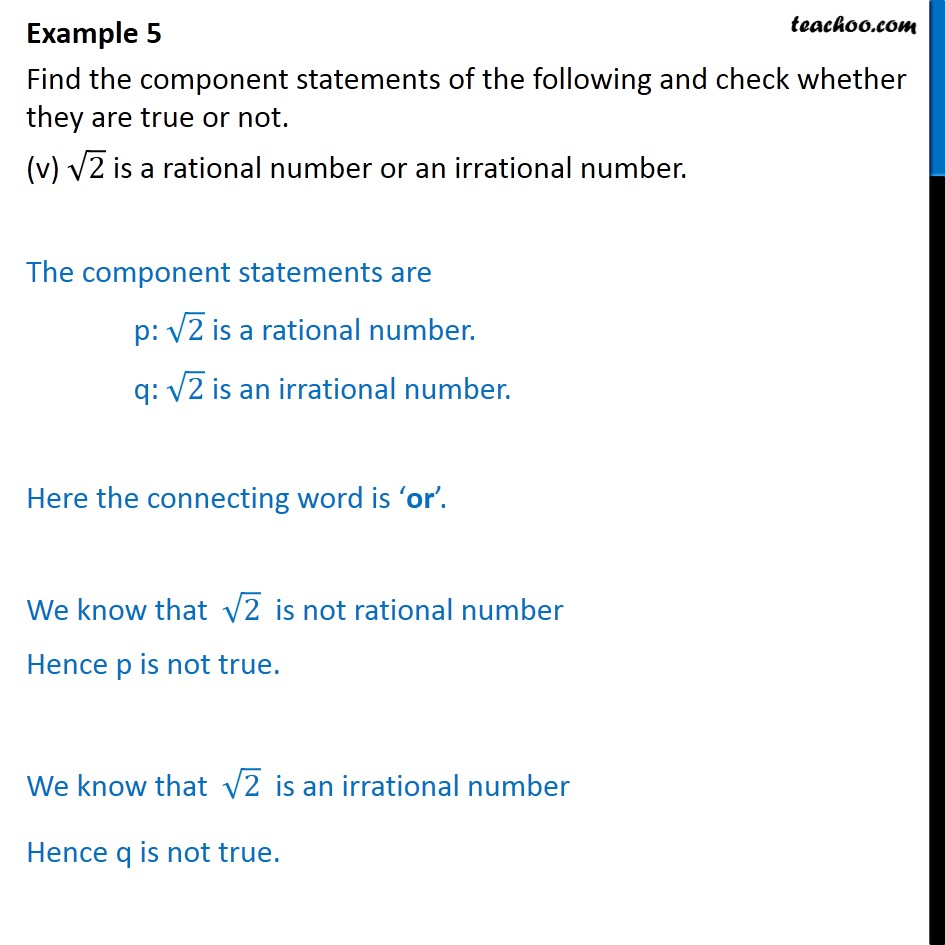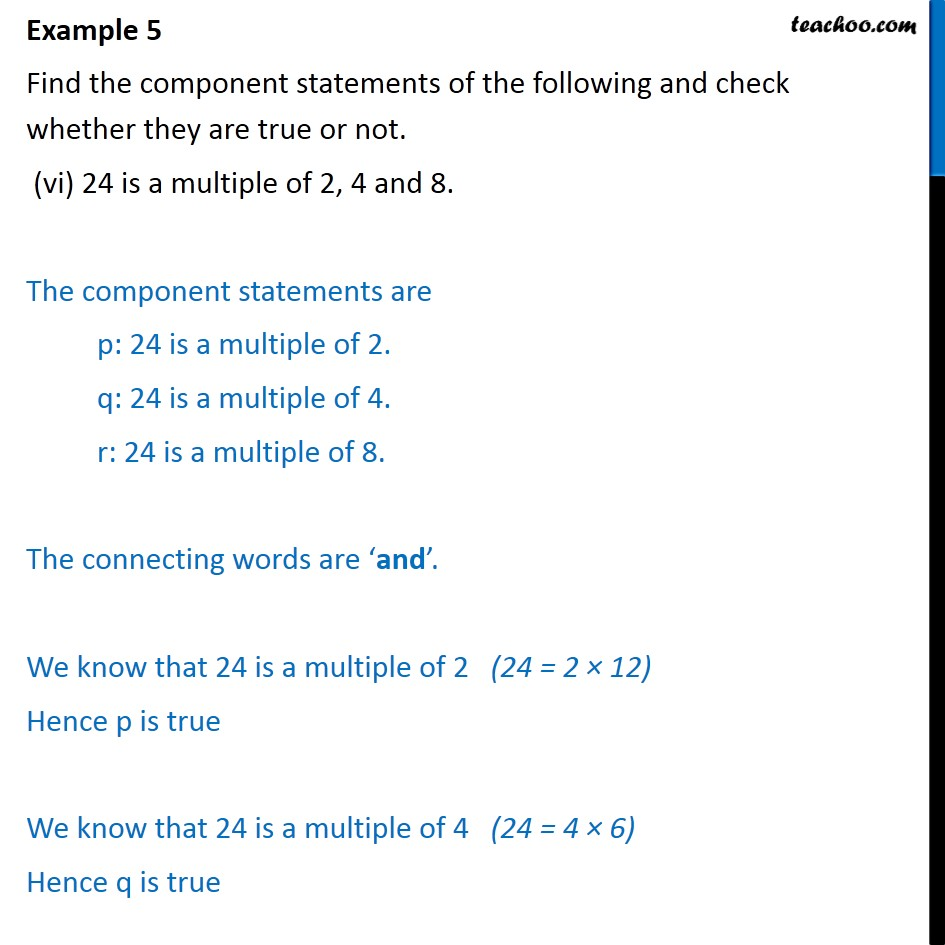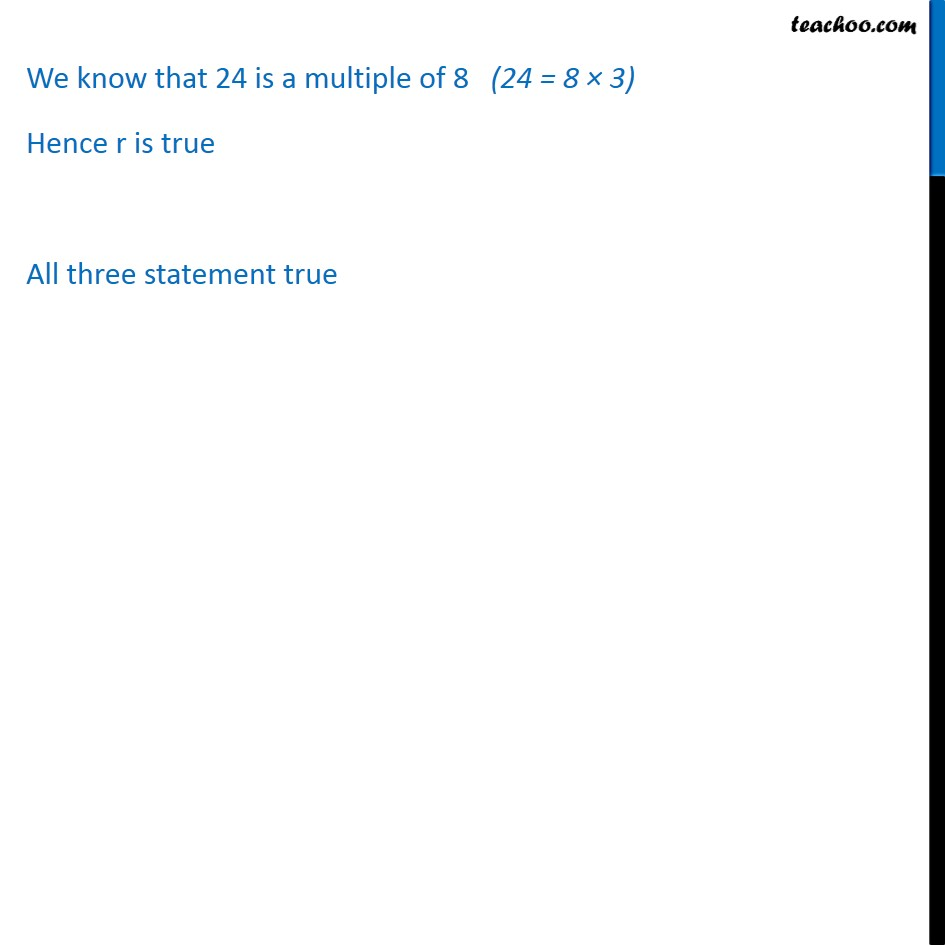1. Class 11
2. Important Question for exams Class 11
3. Chapter 14 Class 11 Mathematical Reasoning

Transcript

Example 5 Find the component statements of the following and check whether they are true or not. (i) A square is a quadrilateral and its four sides equal. The component statements are p: A square is quadrilateral. q: A square has all its sides equal. The connecting word is And . We know that a square is quadrilateral Hence p is true We know that square has all its equal Hence q is true Hence both component statement are true Example 5 Find the component statements of the following and check whether they are true or not. (ii) All prime numbers are either even or odd. The component statements are p: All prime numbers are odd numbers. q: All prime numbers are even numbers. The connecting word is or . We know that All prime number are not even Example: 3 is prime not even Hence p is false We know that All prime number are not odd Example: 2 is prime but not odd Hence q is false. Hence ,both the components statement are false. Example 5 Find the component statements of the following and check whether they are true or not. (iii) A person who has taken Mathematics or Computer Science can go for MCA. The component statements are p: A person who has taken Mathematics can go for MCA. q: A person who has taken computer science can go for MCA Here the connecting word is or . We know that A person can for MCA if he has mathematics Hence p is true. We know that a person can go for MCA if he has taken Computer Science Hence q is true. Hence both the component statement are true Example 5 Find the component statements of the following and check whether they are true or not. (iv) Chandigarh is the capital of Haryana and UP. The component statements are p: Chandigarh is the capital of Haryana. q: Chandigarh is the capital of UP. Here the connecting word is and We know that capital of Haryana is Chandigarh Hence p is true. We know that the capital of UP. is not Chandigarh Hence q is false Example 5 Find the component statements of the following and check whether they are true or not. (v) 2 is a rational number or an irrational number. The component statements are p: 2 is a rational number. q: 2 is an irrational number. Here the connecting word is or . We know that 2 is not rational number Hence p is not true. We know that 2 is an irrational number Hence q is not true. Example 5 Find the component statements of the following and check whether they are true or not. (vi) 24 is a multiple of 2, 4 and 8. The component statements are p: 24 is a multiple of 2. q: 24 is a multiple of 4. r: 24 is a multiple of 8. The connecting words are and . We know that 24 is a multiple of 2 (24 = 2 12) Hence p is true We know that 24 is a multiple of 4 (24 = 4 6) Hence q is true We know that 24 is a multiple of 8 (24 = 8 3) Hence r is true All three statement true

Chapter 14 Class 11 Mathematical Reasoning

Class 11
Important Question for exams Class 11